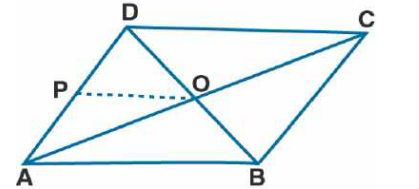# ML Aggarwal Solutions Class 9 Mathematics Solutions for Mid Point Theorem Exercise 11 in Chapter 11 - Mid Point Theorem

. The diagonals AC and BD of a parallelogram ABCD intersect at O. If P is the midpoint of AD, prove

that

(i) PQ || AB

(ii) PO = ½ CD

It is given that

ABCD is a parallelogram in which diagonals AC and BD intersect each other

At the point O, P is the midpoint of AD

Join OP

To find: (i) PQ || AB (ii) PQ = ½ CD \Proof:

(i) In parallelogram diagonals bisect each other

BO = OD

Here O is the mid-point of BD

In Δ ABD

P and O is the midpoint of AD and BD

PO || AB and PO = ½ AB ….. (1)

Hence, it is proved that PO || AB.

(ii) ABCD is a parallelogram

AB = CD ……. (2)

Using both (1) and (2)

PO = ½ CD

Related Questions
Exercises

Lido

Courses

Teachers

Book a Demo with us

Syllabus

Maths
CBSE
Maths
ICSE
Science
CBSE

Science
ICSE
English
CBSE
English
ICSE
Coding

Terms & Policies

Selina Question Bank

Maths
Physics
Biology

Allied Question Bank

Chemistry
Connect with us on social media!